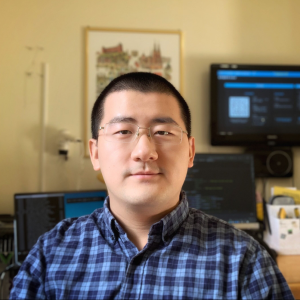# Natural Computation Methods for Machine Learning Note 14

2020年3月25日 4005点热度 0人点赞 0条评论

# Natural Computation Methods for Machine Learning Note 14

## Swarm Intelligence

Intelligence <- Social behavior

• Intelligence can emerge from social interaction.
• Emergent behaviour – when a group behaves in ways that were not ”programmed” into its members

• Swarm intelligence

• simulated social interaction
• emergent collective intelligence in groups of simple agents

## Bird flocks and fish schools

• Local interaction
• Simple local rules – a weighted combination of several goals
• match velocity of neighbours
• avoid collisions with neighbours
• avoid getting too far from neighbours
• Sufficient to make very realistic simulations
• used in movies and computer graphics
• remove the match-velocity rule: insect swarm
• remove collision rule: cultural interaction

## Ants

• Individual ants can not find shortest route. The colony does.
• Ant colonies are much more intelligent than ants.
• Ant colonies build cognitive map in their environment, through pheromone trails.

## Stigmergy ❗❗❗

Indirect communication and coordination, by local modification and sensing of the environment.

## Computational tools

• Cellular automata
• Ant Colony Optimization
• Particle Swarm Optimization

### Cellular automata

• Massively parallel system of identical communicating state machines (cells).
• A cell’s state (e.g. on/off) is a function of the states of it communicates with (its neighbours).
• Used to model/animate fluids (e.g. the water in Find Nemo), gases, bacterial growth, swaying grass, social interaction, epidemics, in ecological simulations etc.

application:

• Construction and repair
• Collaborating simple robots
• Modelling
• Water, avalanches, traffic flows
• Map/level generators for games

### Ant Colony Optimization

Family of combinatorial optimization algorithms, based on ant behaviour

Common benchmark: the Travelling Salesman Problem (TSP)

Common ’real’ applications: Scheduling and Network routing (AntNet)

Members: ACS, Ant-Q, MMAS, ASrank,

• most of which are extensions to Dorigo’s Ant
System (AS)

#### Traveling Salesman Problems (TSP)

• Find the shourtest tour through N cities, and then back to the starting point, such that each city is visited once and only once.
• NP-hard
• (N-1)!/2 possible tours
• exhaustive search intractable
• Specialized algorithms exist
• and are hard to beat

#### Ant System for TSP

Each ant (k)

• is placed in a randomly selected city
• remembers the partial solution found so far (initially, the start city only)
• moves stochastically from city (i) to city (j), by some transition probability p_{i j}^{k}(t)

which depends on

• pheromone intensity, \tau_{i j}
• local information, \eta_{i j} (distance)
• whether j is feasible (not already visited)

#### Transition probabilities#### Effects of \alpha and \beta

p_{i j}^{k}(t)=\frac{\left[\tau_{i j}(t)\right]^{\alpha} *\left[\eta_{i j}\right]^{\beta}}{\sum_{c \in C_{i}^{k}}\left[\tau_{i c}(t)\right]^{\alpha} *\left[\eta_{i c}\right]^{\beta}}, j \in C_{i}^{k}

• If a=0, b>0
• Pheromone information discarded, only local info used
• Stochastic greedy search with multiple starting points
• If a>0, b=0
• No local information used, only pheromones
• May lead to premature convergence

#### Pheromone update

When all ants have completed a tour, let each
ant deposit pheromones on the paths it followed

\tau_{i j}(t+1)=(1-\rho) \tau_{i j}(t)+\sum_k \Delta \tau_{k}{i j}^{k}(t) \quad \forall(i, j)

\rho Evaporation rate(蒸发率) 1<\rho\leq 1

k Sum over all ants, k

\Delta \tau_{i j}^{k}(t)=\left{\begin{array}{cl}1 / L_{k}(t)&\text { if path ij was used by ant } k\\0&\text { otherwise }\end{array}\right.

L_{k}(t)= length of ant k’s tour

#### Ant Colony Opt

• ACO is most promising for non-stationary
problems (e.g. network routing)
• The TSP solution demonstrated here
works, but is not state-of-the-art

### What is "optimal"?

This is different from other course

In practice, optimality includes other factors as well

• time and cost to setup (not only to run)
• amount of knowledge required
• sufficiently good is often good enough

It is often better to use an algorithm/method you know well,
than to search for (and tune) the "best" one.
But, of course, if you happen to know the best one ...Dong Wang

I will work as a PhD student of TU Graz in Austria. My research interests include Embedded/Edge AI, federated learning, computer vision, and IoT.